# The Pythagorean Theorem Lesson Plan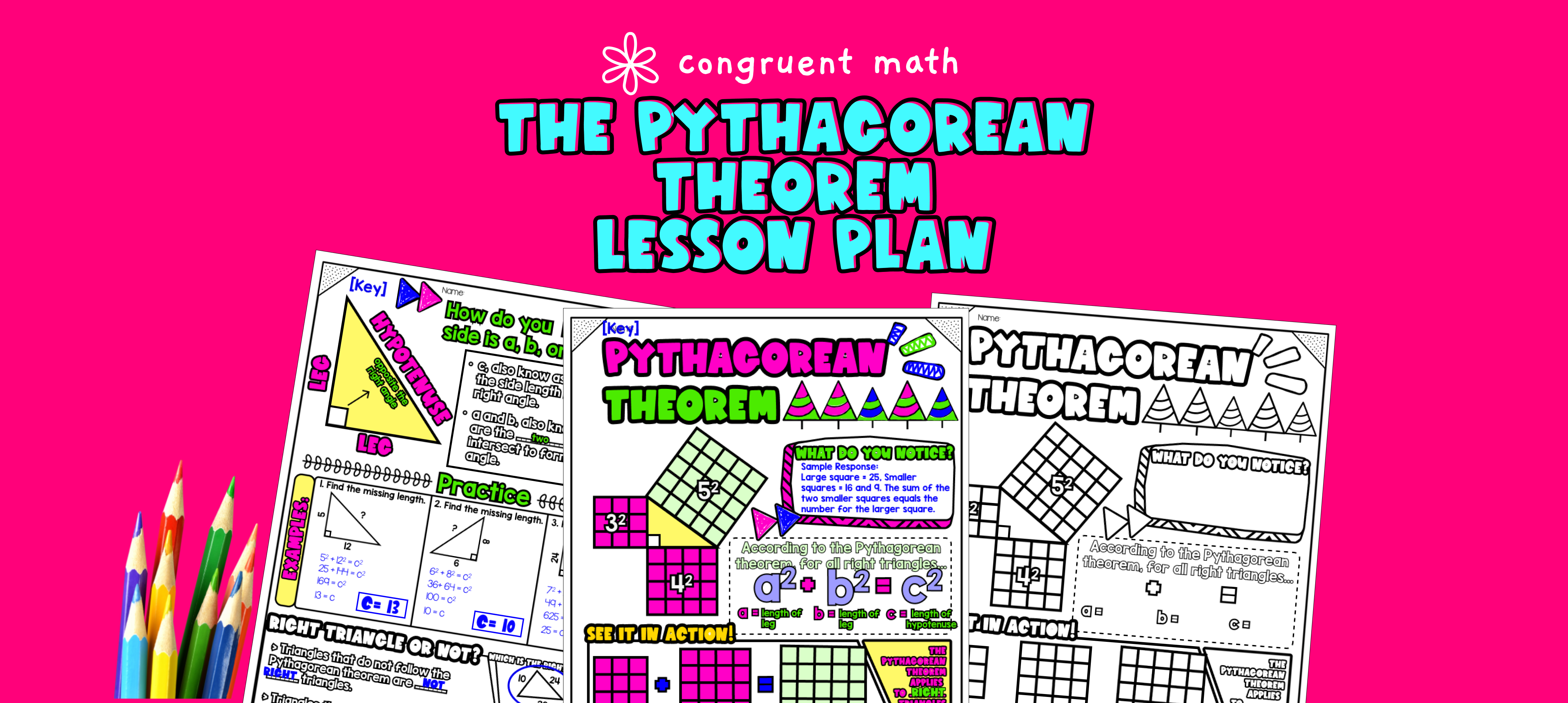Pin This

## Overview

Ever wondered how to teach the Pythagorean Theorem in an engaging way to your 8th grade students?

In this lesson plan, students will learn about the Pythagorean Theorem proofs, legs and hypotenuse, right triangles, and their real-life applications. Through artistic, interactive guided notes, check for understanding, a color by code activity, and a real-life application example, students will gain a comprehensive understanding of the Pythagorean Theorem.

The lesson culminates with an exploration of baseball diamond highlighting the importance of understanding the Pythagorean Theorem in real-world scenarios.

## Get the Lesson Materials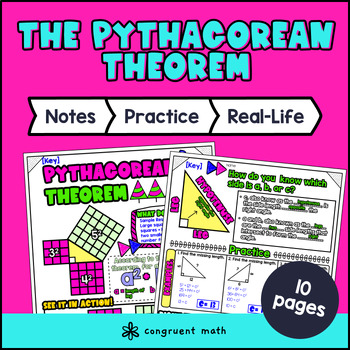\$4.25

## Learning Objectives

After this lesson, students will be able to:

• Identify the hypotenuse and legs of a right triangle

• Use the Pythagorean Theorem to find the length of a missing side in a right triangle

• Apply the Pythagorean Theorem to solve real-life problems

## Prerequisites

Before this lesson, students should be familiar with:

• Basic understanding of triangles (including how to identify right triangles) and their properties, such as side lengths and angle measures

• Basic algebra skills, including solving for unknown variables and simplifying equations

• Familiarity with squaring and square roots

## Key Vocabulary

• Pythagorean Theorem

• Hypotenuse

• Legs

• Right triangle

• Square Roots

## Procedure

### Introduction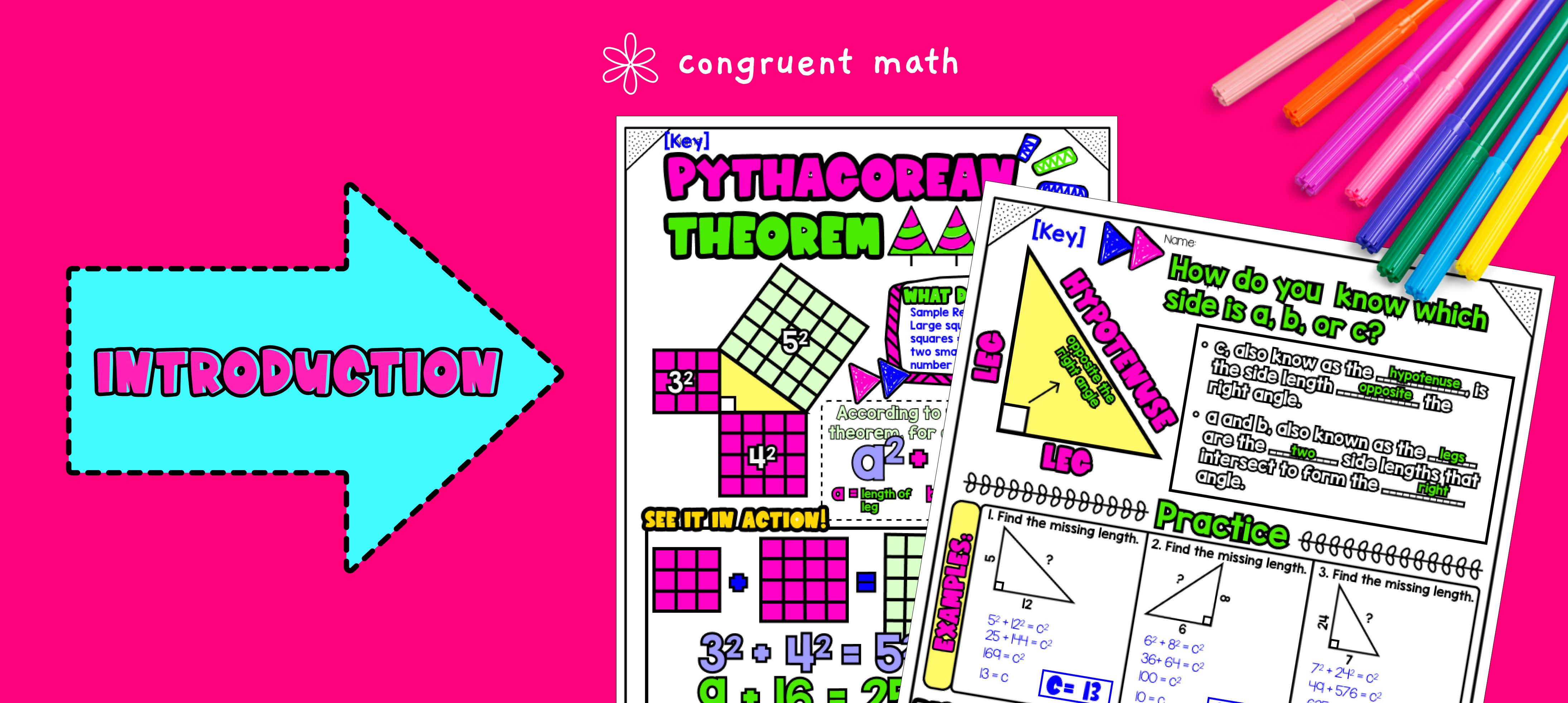Pin This
• As a hook, ask students why the Pythagorean Theorem is important in real life, such as finding the dimensions of a baseball diamond. Use the guided notes to introduce the Pythagorean Theorem.

• Walk through the key points of the topic of the guided notes to teach. Refer to the FAQs for a walk-through on this, as well as ideas on how to respond to common student questions.

• For Page 1 of the guided notes, introduce the Pythagorean Theorem with the worked example "what do you notice."

• For Page 2 of the guided notes, explain how to know which side is a, b, or c, and check for understanding. Based on student responses, reteach concepts that students need extra help with.

### Practice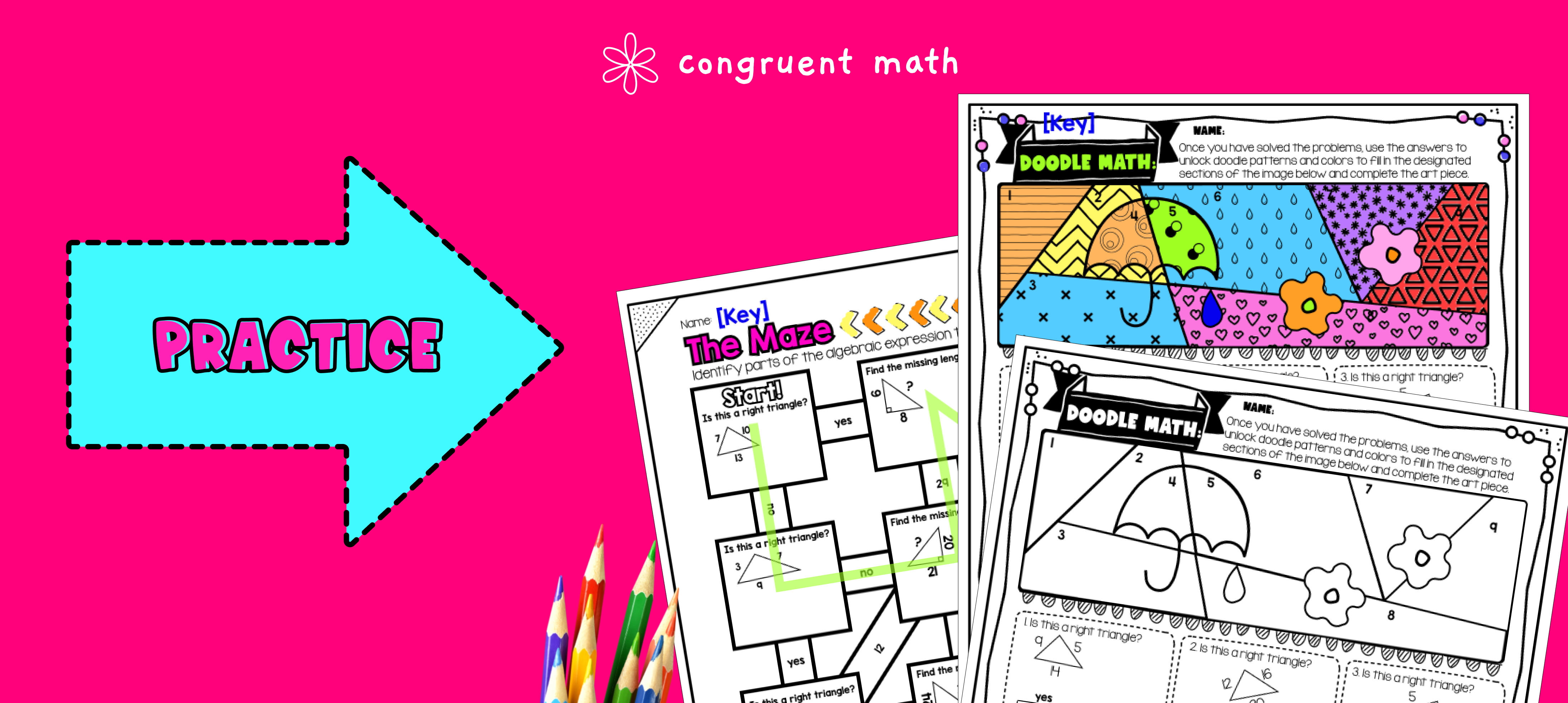Pin This
• Have students practice using the Pythagorean Theorem with the Maze activity. Walk around to answer student questions.

• Fast finishers can dive into the Doodle Math activity for extra practice. You can assign it as homework for the remainder of the class.

### Real-Life Application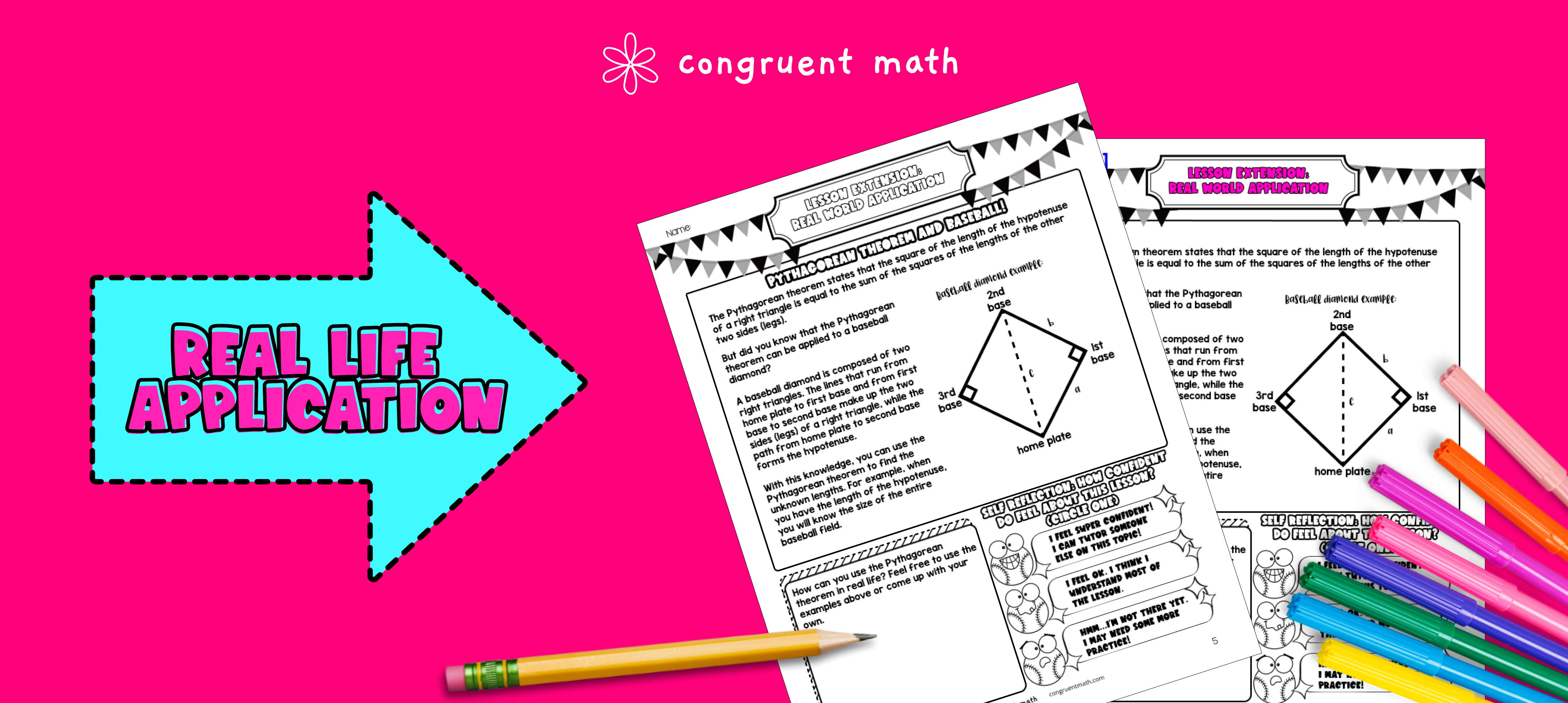Pin This
• Bring the class back together, and introduce the concept of using the Pythagorean Theorem to determine the dimensions of a baseball diamond. Explain that the Pythagorean Theorem can be used to find the distance between any two points, even if they are not directly next to each other. In the case of a baseball diamond, the distance from home plate to first base, home plate to second base, and so on can be found by using the Pythagorean Theorem. Refer to the FAQ for more ideas on how to teach it!

## Extensions

### Hands-on project

A fun, no-prep way for students to practice is my Pythagorean Spiral project. Students will use a square to draw successive triangles and create a spiral. They will then measure the spiral and solve for the length of the hypotenuse for every triangle in their Pythagorean spiral. At the end of the activity, students can turn their Pythagorean spiral into an art project.